Kepler: Area of a circle

Kepler wrote Nova stereometria doliorum vinariorum (New solid geometry of wine barrel) in 1615. This book is a systematic work on the calculation of areas and volumes by infinitesimal techniques. Kepler opens his book with the problem of determining the area of the circle. He regarded the circle as a regular polygon with an infinite number of sides, and its area is made up of infinitesimal triangles. (See Boyer, p. 108)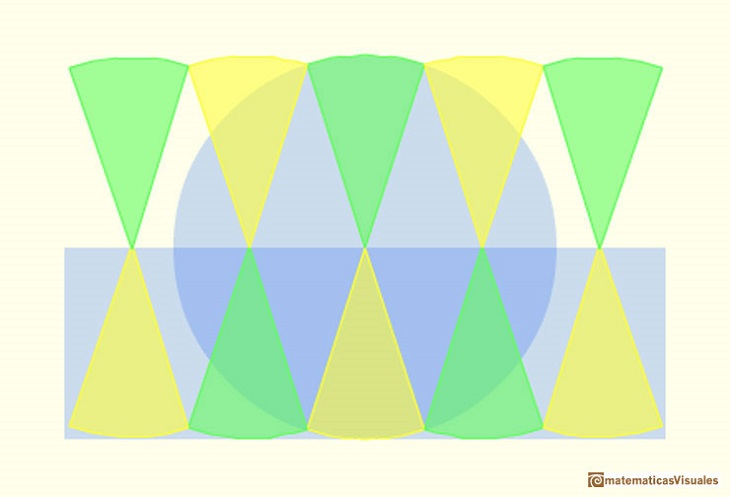The perimeter of a circle is called the circunference. If r is the radius of the circle and d is the diameter we can write the circunference as: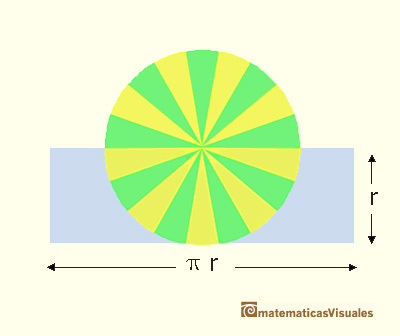then we can deduce the area of the circle:

Margaret Baron wrote about this aspect of Kepler's work: "Of the circle he says that the circunference has as many parts as it has points, each part forming the base of an isosceles triangle with vertex at the center of the circle. The circle is accordingly made up of an infinity of small triangles, each with its base on the circunference and with altitude equal to the radius of the circle. Replacing these triangles by a single triangle with the circunference as base the area of the circle is thus given in terms of the circunference and radius." (Baron, p. 110).

This is the original drawing in Kepler's book. We can read this page, and the full book, in The Posner Memorial Collection,Carnegie Mellon University LibrariesREFERENCES

Margaret E. Baron, The Origins of the infinitesimal Calculus, Dover Publications, New York, 1987.
Carl B. Boyer,The History of the Calculus and its conceptual development, Dover Publications, New York, 1959 (p. 108)In his book 'On Conoids and Spheroids', Archimedes calculated the area of an ellipse. It si a good example of a rigorous proof using a double reductio ad absurdum.Transforming a circle we can get an ellipse (as Archimedes did to calculate its area). From the equation of a circle we can deduce the equation of an ellipse.This perpective projection is area-preserving. If we know the surface area of a sphere we can deduce the volume of a sphere, as Archimedes did.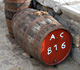Studying the volume of a barrel, Kepler solved a problem about maxima in 1615.Using Cavalieri's Principle we can calculate the volume of a sphere.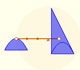Archimedes show us in 'The Method' how to use the lever law to discover the area of a parabolic segment.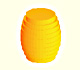Kepler was one mathematician who contributed to the origin of integral calculus. He used infinitesimal techniques for calculating areas and volumes.